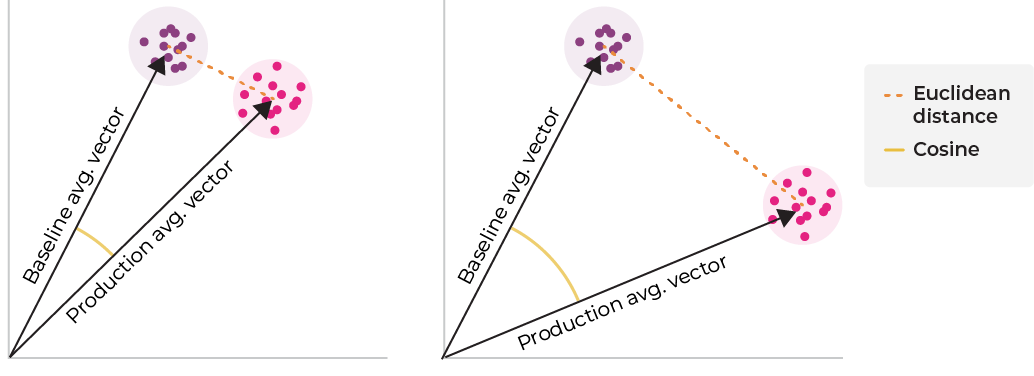# Embedding Drift

Track change for unstructured data

### What is Embedding Drift

The challenge with measuring unstructured data drift is that you need to understand the change in relationships inside the unstructured data itself. Drift helps you understand this.

#### Examples of what Drift can identify:

1. 1.
New set of images you didn't train on shows up in production
2. 2.
New entities showing up in a new version of the model
3. 3.
Issues with data quality changes (blurry, spotted, lightened, darkened, rotated, or cropped images)
4. 4.
Changes in terminology in the data or changes to the context or meaning of words

### How is Embedding Drift Calculated

Once you set up a baseline, Arize compares embedding vectors between different periods of time to determine the occurrence of drift. To do so, Arize computes the Euclidean distance between the primary dataset’s centroid and the baseline's, thus allowing you to detect if drift has happened and when it has occurred.

#### Example Scenario:

Let us have two samples of vectors:
• Sample A: [1, 2, 3]; [4, 5, 6]; [7, 8, 9] .
• Sample B: [-1, 2,4]; [11, 6, 0]
The centroid vectors are: [4, 5, 6], and [5, 4, 2], respectively. We then calculate the Euclidean Distance as follows:
$\sqrt{(x_1 - y_1)^2 + (x_2 - y_2)^2 + (x_3 - y_3)^2}=\sqrt{(4-5)^2+(5-4)^2+(6-2)^2}\approx4.24$The above picture shows two groups of vectors –- one for the baseline, and the other for production. Both euclidean and cosine distances are greater when the two vectors are further apart. This is monitored as the embedding drift.

### What do you need to calculate Drift?

Arize uses the raw embedding vectors it receives to track the drift of your unstructured input data. To calculate the Euclidean distance, we need two sets of data:
• Primary Dataset -> The dataset we will measure drift on. This can be any dataset that you have ingested into Arize
• Baseline --> Defaults to the configured model baseline. This is what we will compare the Primary Dataset toChoose from the drop downs at the top of the page to adjust you primary and baseline datasets.
Note: The grey bars in the above image are the data traffic. Low volumes of data are not reliable for calculating drift. Try changing the time range or adding more data.

### Using Arize to Track Embedding Drift

Generally speaking, when your Euclidean distance is low, there is a strong overlap between the production and baseline datasets. When problematic or new data is introduced into the dataset, the Euclidean distance will increase indicating that drift has occurred. We can take this one step further by generating the UMAP to visualize these differences in embeddings.Drift in dataset
In the example above, we can see (in the plot on the top of the page) that there's a week where there's an increase in this distance, signaling a drift on the input dataset that requires further investigation. In other words, this shows that during that time, the production data that was sent to our model was different than the model baseline.

### Set up an Embedding Drift Monitor

You can also set up drift monitors to track embedding drift. This allows you to automate drift tracking and receive alerts when your embeddings have drifted. See Drift Monitors for more details.